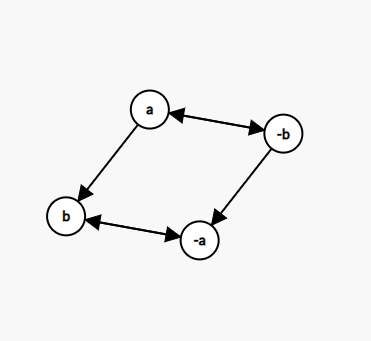2018-08-22 19:51:06

## 什么是 2-SAT？

• Anguei: 我要求代码当中满足下列条件之一：
1. 不写 using namespace std; （ $\neg a$）
2. 使用读入优化 （$b$）
3. 大括号不换行 （$\neg c$）
• Anfangen: 我要求代码当中满足下条件之一：
1. using namespace std; （$a$）
2. 使用读入优化 （$b$）
3. 大括号不换行 （$\neg c$）
• Zachary_260325：我要求代码当中满足下条件之一：
1. 不写 using namespace std; （$\neg a$）
2. 使用 scanf （$\neg b$）
3. 大括号换行 （$c$）

Q: 这可怎么赋值啊？暴力？

A: 对，这是 SAT 问题，已被证明为 NP 完全 的，只能暴力。

Q: 那么 2-SAT 是什么呢？

A: 2-SAT，即每位同学 只有两个条件（比如三位同学都对大括号是否换行不做要求，这就少了一个条件）不过，仍要使所有同学得到满足。于是，以上布尔方程当中的 $c,\neg c$ 没了，变成了这个样子：$(\neg a\vee b) \wedge (a\vee b) \wedge (\neg a\vee\neg b)$

## 怎么求解 2-SAT 问题？

$\neg a\vee b$ $a\rightarrow b\wedge\neg b\rightarrow\neg a$
$a \vee b$ $\neg a\rightarrow b\wedge\neg b\rightarrow a$
$\neg a\vee\neg b\space \space$ $a\rightarrow\neg b\wedge b\rightarrow\neg a$## 说了这么多，咋不上代码啊？

### 建图

n = read(), m = read();
for (int i = 0; i < m; ++i) {
// 笔者习惯对 x 点标号为 x，-x 标号为 x+n，当然也可以反过来。
if (va && vb) { // a, b 都真，-a -> b, -b -> a
g[a + n].push_back(b);
g[b + n].push_back(a);
} else if (!va && vb) { // a 假 b 真，a -> b, -b -> -a
g[a].push_back(b);
g[b + n].push_back(a + n);
} else if (va && !vb) { // a 真 b 假，-a -> -b, b -> a
g[a + n].push_back(b + n);
g[b].push_back(a);
} else if (!va && !vb) { // a, b 都假，a -> -b, b -> -a
g[a].push_back(b + n);
g[b].push_back(a + n);
}
}

n = read(), m = read();
for (int i = 0; i < m; ++i) {
g[a + n * (va & 1)].push_back(b + n * (vb ^ 1));
g[b + n * (vb & 1)].push_back(a + n * (va ^ 1));
}

### 找环

// 注意所有东西都要开两倍空间，因为每个变量存了两次
void tarjan(int u) {
low[u] = dfn[u] = ++dfsClock;
stk.push(u); ins[u] = true;
for (const auto &v : g[u]) {
if (!dfn[v]) tarjan(v), low[u] = std::min(low[u], low[v]);
else if (ins[v]) low[u] = std::min(low[u], dfn[v]);
}
if (low[u] == dfn[u]) {
++sccCnt;
do {
color[u] = sccCnt;
u = stk.top(); stk.pop(); ins[u] = false;
} while (low[u] != dfn[u]);
}
}
// 笔者使用了 Tarjan 找环，得到的 color[x] 是 x 所在的 scc 的拓扑逆序。
for (int i = 1; i <= (n << 1); ++i) if (!dfn[i]) tarjan(i);

### 输出

for (int i = 1; i <= n; ++i)
if (color[i] == color[i + n]) { // x 与 -x 在同一强连通分量内，一定无解
puts("IMPOSSIBLE");
exit(0);
}
puts("POSSIBLE");
for (int i = 1; i <= n; ++i)
print((color[i] < color[i + n])), putchar(' '); // 如果不使用 Tarjan 找环，请改成大于号
puts("");
• star
首页